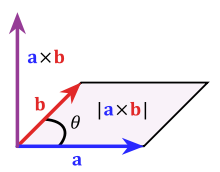1232. 缀点成线

# 1232. 缀点成线#

• 标签：几何、数组、数学
• 难度：简单

## 解题思路 #• 如果 P × Q = 0，则 P 与 Q 共线，有可能同向，也有可能反向。
• 如果 P × Q > 0，则 P 在 Q 的顺时针方向。
• 如果 P × Q < 0，则 P 在 Q 的逆时针方向。

• 先求出第一个坐标与第二个坐标构成的向量 P。
• 遍历所有坐标，求出所有坐标与第一个坐标构成的向量 Q。
• 如果 P × Q ≠ 0，则返回 False。
• 如果遍历完仍没有发现 P × Q ≠ 0，则返回 True。

## 代码 #

  1 2 3 4 5 6 7 8 9 10 11  class Solution: def checkStraightLine(self, coordinates: List[List[int]]) -> bool: x1 = coordinates - coordinates y1 = coordinates - coordinates for i in range(len(coordinates)): x2 = coordinates[i] - coordinates y2 = coordinates[i] - coordinates if x1 * y2 != x2 * y1: return False return True